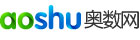奥数呼和浩特站 > 小升初 > 小升初练习题 > 正文

# 五年级奥数之分解质因数运用示例

例1、已知360×A=B×B，其中A、B均为自然数，求A的最小值是几？B的值又为几？

分析与解答：因为 360×A=B2，即为360×A也是一个完全平方数。而 360=5×3×3×2×2×2=(5×3×2)×(3×2×2)，因此可得要使 360×A是一个完全平方数，A的值只能为：5×2=10.所以可得，A的值最小为10.这时B的值为60.

例2、A、B、C均为自然数，已知A×B=132，B×C=156，C×A=143.求A×B×C的值是几？

分析与解答：因为132=11×12，所以A×B =11×12.

156=12×13，所以B×C =12×13.

143=11×13，所以C×A =11×13.

比较以上各式可知，A=11；B=12；C=13.所以A×B×C=11×12×13=1716.

例3、把棱长1厘米的小正方体2100个，堆在一个实心的大长方体，这个长方体的高为10厘米，并且长、宽均大于高，求这个长方体的表面积。

分析与解答：根据题中的条件可知，这个长方体的体积为2100立方厘米，因为长方体的高为10厘米，所以长方体的底面积为：2100÷10=210(平方厘米)。又因为长方体的长、宽均大于10.而210=2×5×3×7=(3×5)×(2×7)=15×14.因此可得，这长方体的长为15厘米，宽为14厘米，高为10厘米。它的表面积为：(15×14+15×10+14×10)×2=1000(平方厘米)。

例4、把一个长16厘米，宽为8厘米，高为4厘米的长方体锯成若干个小正方体，然后拼成一个大正方体，求这个大正方体的表面积。

分析与解答：因为将一个长方体锯成若干个小正方体后拼成的大正方体的体积同原来的长方体的体积是相等的。长方体的体积为：16×8×4=512(立方厘米)。而 512=2×2×2×2×2×2×2×2×2=8×8×8.所以可知，大正方体的棱长为8厘米。大正方体的表面积为：8×8×6=384(平方厘米)。

例5、两个自然数的乘积是2835，它们的最大公因数是9，求这两个数。

分析与解答：因为两个数的最大公因数是9，因此可知这两个数中都有因数9.因为2835=5×7×9×9=45×63.所以可知这两个自然数分别为45和63.

例6、1×2×3×4×5×…×99×100的积，末尾有多少个连续的零？

分析与解答：因为2×5=10，这样含有一个2和一个5，乘积末尾就会有一个0.因此，只要观察这100个因数中一共含有多少个2和5.又知，在这100个因数中，含2个的数一定多于5的个数，所以只需知道乘积中含有5的个数，就可知积的末尾连续0的个数。

这100个因数中是5的倍数的有5、10、15……95、100共有20个，其中25、50、75、100又是25的倍数，各有两个5.所以乘积中共有5的个数是20+4=24(个)。因此，乘积的末尾共有24个连续的0.

例7、有四个小朋友的年龄一个比一个大一岁，他们的年龄的积是5040，求他们各是多大？

分析与解答：把5040分解质因数，得5040=2×2×2×2×3×3×5×7，然后组合：7，2×2×2=8，3×3=9，2×5=10

例8、甲数比乙数大9，两数的积是1620，求这两个数。

分析与解答：1620=22×34×5=(32×22)×(32×5)

甲数是45，乙数是36.

例9、把14、30、33、75、143、169、4445、4953分成两组，每组四个数且积相等，求这两组数。

分析与解答：八个数的积等于(2×7)×(2×3×5)×(3×11)×(3×5×5)×(11×13)×(13×13)×(5×7×127)×(3×13×127)。

在积中共有质因数2(2个)，3(4个)，5(4个)，7(2个)，11(2个)，13(4个)，127(2个)

分组为：

A组：　　　　　　　　B组：

4445=5×7×127　　　4953=3×13×127

169=13×13　　　　 143=11×13

33=3×11　　　　　 14=2×7

30=2×3×5　　　　　75=3×5×5

例10、1*2*3*4*5*6*……*a的积的末尾连续有20个0，a最小是多少，最大是多少？

分析与解答：n！末尾零的个数等于 f(n！) = [n/5] + [n/5^2] + [n/5^3] + [n/5^4] + ……，其中[]为取整运算85到89的末尾是20个零。